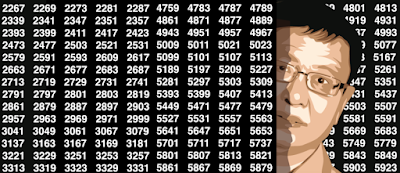## Monday, July 10, 2017

### How to Detect if Numbers are Random or Not

In this article, you will learn some modern techniques to detect whether a sequence appears as random or not, whether it satisfies the central limit theorem (CLT) or not -- and what the limiting distribution is if CLT does not apply -- as well as some tricks to detect abnormalities.  It leads to the exploration of time series with massive, large-scale (long term) auto-correlation structure, as well as model-free, data-driven statistical testing. No statistical knowledge is required: we will discuss deep results that can be expressed in simple English. Most of the testing involved here uses big data (more than a billion computations) and data science, to the point that we reached the accuracy limits of our machines.  So there is even a tiny piece of numerical analysis in this article.
Potential applications include testing randomness, Monte Carlo simulations for statistical testing, encryption, blurring, and steganography (encoding secret messages into images) using pseudo-random numbers. A number of open questions are discussed here, offering the professional post-graduate statistician new research topics both in theoretical statistics and advanced number theory. The level here is state-of-the-art, but we avoid jargon and some technicalities to allow newbies and non-statisticians to understand and enjoy most of the content.  An Excel spreadsheet, attached to this document, summarizes our computations and will help you further understand the methodology used here.
Interestingly, I started to research this topic by trying to apply the notorious central limit theorem (CLT) to non-random (static) variables -- that is, to fixed sequences of numbers that look chaotic enough to simulate randomness. Ironically, it turned out to be far more complicated than using CLT for regular random variables. So I start here by describing what the initial CLT problem was, before moving into other directions such as testing randomness, and the distribution of the largest gap in seemingly random sequences.  As we will see, these problems are connected.

### Machine Learning Perspective on the Twin Prime Conjecture

This article focuses on the machine learning aspects of the problem, and the use of pattern recognition techniques leading to interesting,...